# C++ Linked Lists

The term 'list' refers to a linear collection of data. One form of linear lists are arrays. As you know that the linear relationship between the data elements of array is reflected by the physical relationship of the data in the memory (i.e., sequential allocation) no by any information contained in the data elements themselves. However, a list can also be stored as having data elements pointing to the next in the sequence (i.e., linked allocation) i.e., a linked list.

Linked Lists is a linear collection of data elements, called nodes pointing to the next nodes by means of pointers. Each node is divided into the two parts:

• the first part containing the information of the element
• the second part called the link or next pointer containing the address of the next node in the list

## Need for Linked Lists

The simplest one of data structures i.e., an array can be used only when its number of elements along with element sizes are predetermined, since the memory is reserved before processing. For this reason, arrays are called dense lists or static data structures. Now consider a situation where exact number of elements are not known. In such a case, estimates might go wrong. Also during processing i.e., either more memory is required or extra free spaces lie in the array. Another problem associated with arrays is complexity involved in insertions and deletions of elements.

## Memory Allocation (Dynamic Vs. Static)

Each data elements, stored in the memory, is given some memory. This processes of giving memory is called memory allocation. The memory (main memory) can be allocated in two manners : dynamically and statically.

### Static Memory Allocation

Static Memory Allocation techniques reserves fixed amount of memory before actual processing takes place, therefore, number of elements to be stored must be predetermined. Such type of memory allocation is called static memory allocation. Arrays are allocated memory using Static Memory Allocation technique only.

### Dynamic Memory Allocation

Dynamic Memory Allocation technique facilitates allocation of memory during the program execution itself, as and when required. This technique of memory allocation is called dynamic memory allocation. Dynamic memory allocation also facilitates release of memory, if memory is not required any more. Data structures like linked lists and trees use this very technique for their memory allocation.

## Singly Linked Lists

The one-way implementation of a general list having elements : 'linked', 'lists', 'are', 'data', 'structures' can be done as shown below :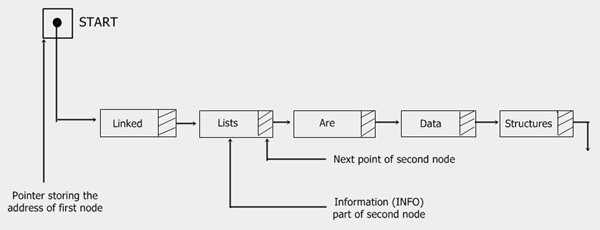The pointer START is a special pointer which stores the very first address of a linked list. The Next pointer of the last node stores NULL value (shown as earth sign), which means this node is not pointing to any other node i.e., it is the last node in the list.

## C++ Linked Lists Example

Let's take some examples for complete understanding on the concept of linked lists in C++ practically. Here the programs listed are:

• Insertion in the beginning of a list
• Insertion in the end of a list
• Deletion from the beginning of a list
• Traversal in a list

### Insertion in the beginning of a List

In this program

• The pointer start, points to the beginning of the list
• Function create_new_node() takes one integer argument, allocates memory to create a new node and returns the pointer to the new node. (return type: node *)
• Function insert_at_beg() takes node * type pointer as argument and inserts this node in the beginning of list.
• Function display() takes node * type pointer as argument and displays the list from this pointer till the end of the list.
```/* C++ Linked Lists - Example Program of Linked Lists
* Inserting in the beginning of the list */

#include<iostream.h>
#include<conio.h>
#include<process.h>

struct node
{
int info;
node *next;
} *start, *newptr, *save, *ptr;

node *create_new_node(int);
void insert_at_beg(node *);
void display(node *);

void main()
{
clrscr();
start = NULL;
int inf;
char ch='y';

while(ch=='y' || ch=='Y')
{
clrscr();
cout<<"Enter Information for the new node: ";
cin>>inf;
cout<<"\nCreating new node..!!..Press any key to continue..";
getch();
newptr = create_new_node(inf);
if(newptr != NULL)
{
cout<<"\n\nNew node created successfully..!!\n";
cout<<"Press any key to continue..";
getch();
}
else
{
cout<<"\nSorry..!!..cannot create new node..!!..Aborting..!!";
cout<<"\nPress any key to exit..";
getch();
exit(1);
}
cout<<"\n\nNow inserting this node at the beginning of the list..\n";
cout<<"Press any key to continue..\n";
getch();
insert_at_beg(newptr);
cout<<"\nNode successfully inserted at the beginning of the list.\n";
cout<<"Now the list is:\n";
display(start);
cout<<"\nWant to enter more nodes ? (y/n)..";
cin>>ch;
}
getch();
}

node *create_new_node(int n)
{
ptr = new node;
ptr->info = n;
ptr->next = NULL;
return ptr;
}

void insert_at_beg(node *np)
{
if(start==NULL)
{
start = np;
}
else
{
save = start;
start = np;
np->next = save;
}
}

void display(node *np)
{
while(np != NULL)
{
cout<<np->info<<" -> ";
np = np->next;
}
cout<<"!!\n";
}```

Here are the sample runs of the above C++ program: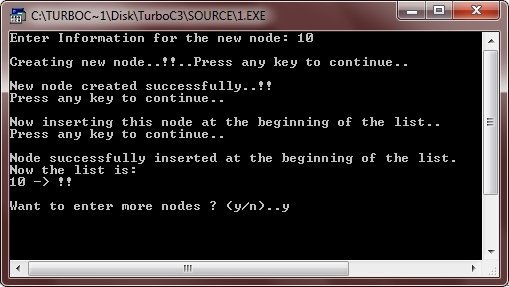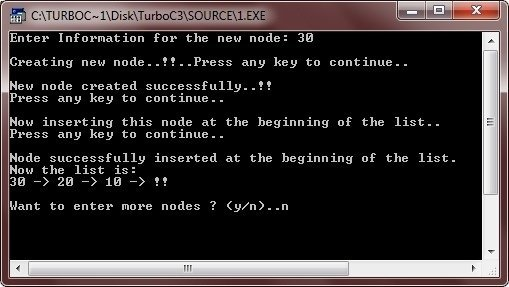### Insertion in the end of a List

In this program

• The start pointer points to the beginning of the list, and the rear points to the last node
• Function create_new_node() takes only one parameter, allocates memory to create a new node and returns the pointer to the new node. (return type: node *)
• Function insert_in_end() takes node * type pointer as argument and inserts this node in the end of the list.
• Function display() takes node * type pointer as argument and displays the list from this pointer till the end of the list.
```/* C++ Linked Lists - Example Program of Linked Lists
* Insertion in the end of the list */

#include<iostream.h>
#include<conio.h>
#include<process.h>

struct node
{
int info;
node *next;
} *start, *newptr, *save, *ptr, *rear;

node *create_new_node(int);
void insert_in_end(node *);
void display(node *);

void main()
{
clrscr();
start = rear = NULL;
int inf;
char ch='y';

while(ch=='y' || ch=='Y')
{
clrscr();
cout<<"Enter Information for the new node: ";
cin>>inf;
cout<<"\nCreating new node..!!..Press any key to continue..";
getch();
newptr = create_new_node(inf);
if(newptr != NULL)
{
cout<<"\n\nNew node created successfully..!!\n";
cout<<"Press any key to continue..";
getch();
}
else
{
cout<<"\nSorry..!!..cannot create new node..!!..Aborting..!!";
cout<<"\nPress any key to exit..";
getch();
exit(1);
}
cout<<"\n\nNow inserting this node in the end of the list..\n";
cout<<"Press any key to continue..\n";
getch();
insert_in_end(newptr);
cout<<"\nNode successfully inserted in the end of the list.\n";
cout<<"Now the list is:\n";
display(start);
cout<<"\nWant to enter more nodes ? (y/n)..";
cin>>ch;
}
getch();
}

node *create_new_node(int n)
{
ptr = new node;
ptr->info = n;
ptr->next = NULL;
return ptr;
}

void insert_in_end(node *np)
{
if(start==NULL)
{
start = rear = np;
}
else
{
rear -> next = np;
rear = np;
}
}

void display(node *np)
{
while(np != NULL)
{
cout<<np->info<<" -> ";
np = np->next;
}
cout<<"!!\n";
}```

Here are some sample runs of the above C++ program: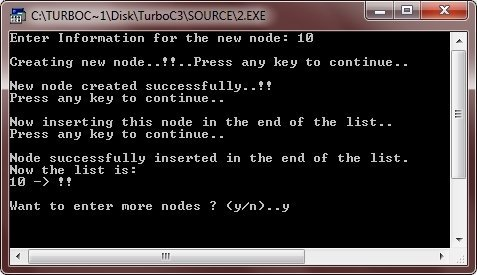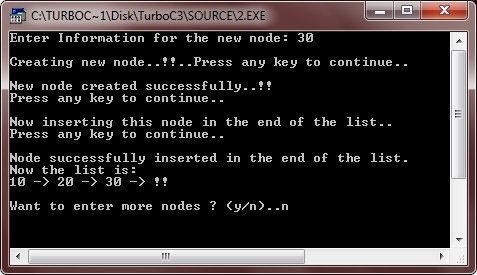### Deletion from the beginning of a List

In this program

• The start pointer points to the beginning of the list and the rear points to the last node
• The function create_new_node() takes one integer argument, allocates memory to create a new node and returns the pointer to the new node. (return type: node *)
• The function insert_node() takes node * type pointer as argument and inserts this node in the end of the list.
• And the function delete_node() deletes a node from the beginning of list being pointed to by the start pointer
```/* C++ Linked Lists - Example Program of Linked Lists
* Deletion from the beginning of the list.
* This program first creates the linked list, then
* allows user to delete nodes from the beginning
* of the list */

#include<iostream.h>
#include<conio.h>
#include<process.h>

struct node
{
int info;
node *next;
} *start, *newptr, *save, *ptr, *rear;

node *create_new_node(int);
void insert_node(node *);
void display_node(node *);
void delete_node();

void main()
{
clrscr();
start = rear = NULL;
int inf;
char ch='y';

while(ch=='y' || ch=='Y')
{
clrscr();
cout<<"Enter Information for the new node: ";
cin>>inf;
newptr = create_new_node(inf);
if(newptr == NULL)
{
cout<<"\nSorry..!!..cannot create new node..!!..Aborting..!!";
cout<<"\nPress any key to exit..";
getch();
exit(1);
}
insert_node(newptr);
cout<<"\nWant to enter more nodes ? (y/n)..";
cin>>ch;
}
clrscr();
do
{
cout<<"The list now is:\n";
display_node(start);
cout<<"\nWant to delete first node ? (y/n)..";
cin>>ch;
if(ch=='y' || ch=='Y');
{
delete_node();
}
}while(ch=='y' || ch=='Y');
getch();
}

node *create_new_node(int n)
{
ptr = new node;
ptr->info = n;
ptr->next = NULL;
return ptr;
}

void insert_node(node *np)
{
if(start==NULL)
{
start = rear = np;
}
else
{
rear -> next = np;
rear = np;
}
}

void delete_node()
{
if(start == NULL)
{
cout<<"Underflow...!!\n";
}
else
{
ptr = start;
start = start->next;
delete ptr;
}
}

void display_node(node *np)
{
while(np != NULL)
{
cout<<np->info<<" -> ";
np = np->next;
}
cout<<"!!\n";
}```

Here are some sample runs of the above C++ program: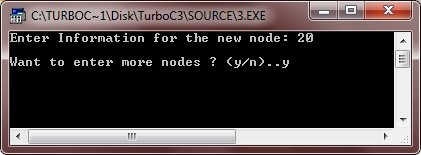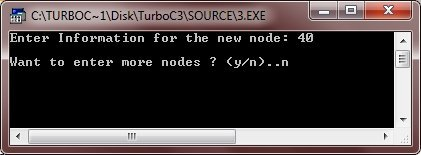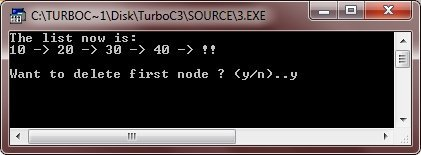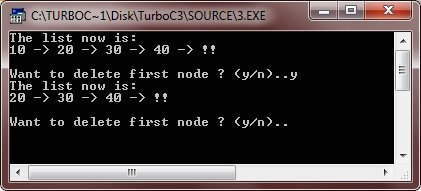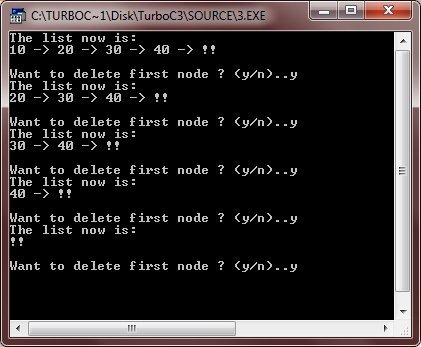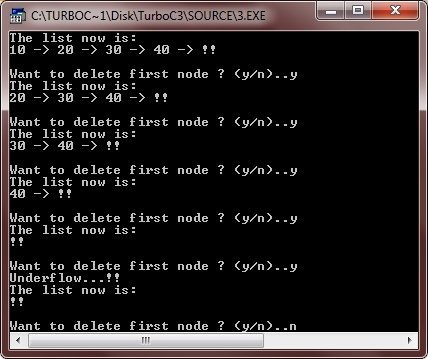### Traversal in a List

In this program

• The start pointer points to the beginning of the list and rear points to the last node
• The function create_new_node() takes one parameter, allocates memory to create a new node and returns the pointer to the new node. (return type: node *)
• The function insert_node() takes node * type pointer as argument and inserts this node in the end of the list.
• And the function traversal() takes node * type pointer as argument and displays the list from this pointer till the end of the list.
```/* C++ Linked Lists - Example Program of Linked Lists
* Traversal in a list */

#include<iostream.h>
#include<conio.h>
#include<process.h>

struct node
{
int info;
node *next;
} *start, *newptr, *save, *ptr, *rear;

node *create_new_node(int);
void insert_node(node *);
void travers(node *);

void main()
{
clrscr();
start = rear = NULL;
int inf;
char ch='y';

while(ch=='y' || ch=='Y')
{
cout<<"Enter Information for the new node: ";
cin>>inf;
newptr = create_new_node(inf);
if(newptr == NULL)
{
cout<<"\nSorry..!!..cannot create new node..!!..Aborting..!!";
cout<<"\nPress any key to exit..";
getch();
exit(1);
}
insert_node(newptr);
cout<<"Want to enter more nodes ? (y/n)..";
cin>>ch;
cout<<"\n";
}
cout<<"The list now is:\n";
travers(start);
getch();
}

node *create_new_node(int n)
{
ptr = new node;
ptr->info = n;
ptr->next = NULL;
return ptr;
}

void insert_node(node *np)
{
if(start==NULL)
{
start = rear = np;
}
else
{
rear -> next = np;
rear = np;
}
}

void travers(node *np)
{
while(np != NULL)
{
cout<<np->info<<" -> ";
np = np->next;
}
cout<<" !!\n";
}```

Here is the sample run of this C++ program.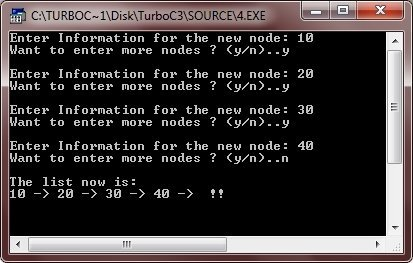Tools
Calculator

Quick Links
Signup - Login - Give Online Test